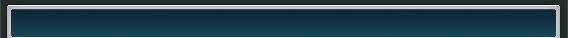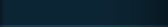Home%    Coherent Noise Detection

function [mode, length_of_histogram] = coherent_noise(histogram, ignore)

%    This routine defines blank, noisy images that have histograms that are not
%    single-moded (e.g. like Gaussian or Poisson noise) as having coherent
%    noise, i.e. artifacts in the image that are not random and not necessrily repetitive.

%   This is useful for determing if an imaging sensor is working properly
%   by imaging a uniform scene/target and confirming that the only signal
%   variations are due to sensor noise.

%   The routine returns the locations of the modes in the histogram
%    Variable "ignore" is the minimum mode height to be recognized.
%    Any frequency of occurrence smaller than this value is considered noise.

length_of_histogram=length(histogram);
for i=1:length_of_histogram-2,
coherent(i)=0;
A=histogram(i+2)-histogram(i+1);
B=histogram(i+1)-histogram(i);

%        If the histogram indicates a fall from a peak and the height of the histogram
%        is high enough, designate this point as a mode

if  ((A<0) & (B>=0)), coherent(i)=1;
end;
if ((coherent==1) & (histogram(i+1)<ignore)), coherent(i)=0;
end;
end;
[mode]=find(coherent);

 Serious Science LLC            Where science fiction becomes fact.